1、通垃圾件和非垃圾训练离线器。

2、经过训练的模型被部署为服务用户的服务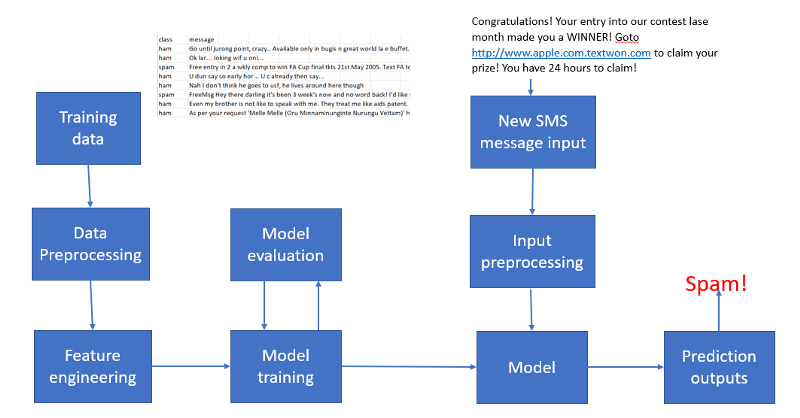import pandas as pd
import numpy as np
from sklearn.feature_extraction.text import CountVectorizer
from sklearn.model_selection import train_test_split
from sklearn.naive_bayes import MultinomialNB
from sklearn.metrics import classification_report

df.drop(['Unnamed: 2', 'Unnamed: 3', 'Unnamed: 4'], axis=1, inplace=True)
df['label'] = df['class'].map({'ham': 0, 'spam': 1})
X = df['message']
y = df['label']
cv = CountVectorizer()
X = cv.fit_transform(X) # Fit the Data
X_train, X_test, y_train, y_test = train_test_split(X, y, test_size=0.33, random_state=42)
#Naive Bayes Classifier
clf = MultinomialNB()
clf.fit(X_train,y_train)
clf.score(X_test,y_test)
y_pred = clf.predict(X_test)
print(classification_report(y_test, y_pred))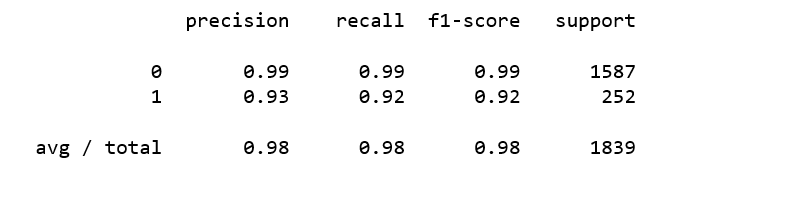Naive Bayes分类器不仅易于实现，而且提供了非常好的性能。在训练模型之后，我们都希望有一种方法来保持模型以供将来使用而无需重新训练。为实现此目的，我们添加以下行以将我们的模型保存为.pkl文件供以后使用。

from sklearn.externals import joblib
joblib.dump(clf, 'NB_spam_model.pkl')

NB_spam_model = open('NB_spam_model.pkl','rb')
clf = joblib.load(NB_spam_model)

spam.csv
app.py
templates/
home.html
result.html
static/
style.css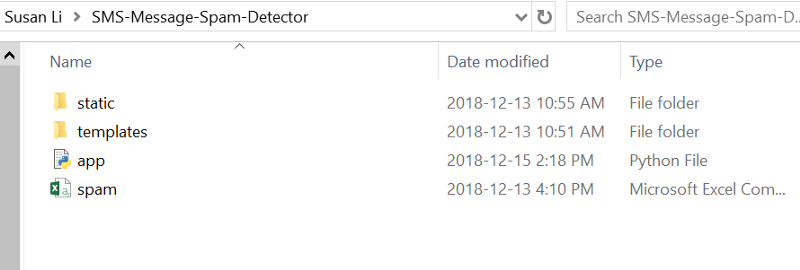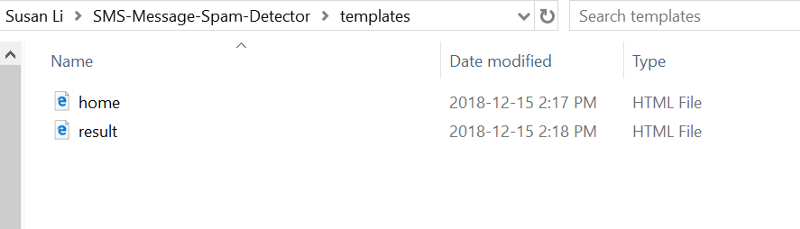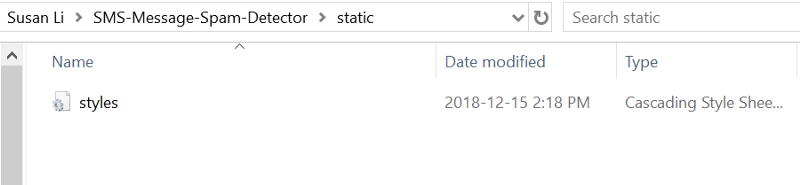app.py

app.py文件包含将由Python解释器执行以运行Flask Web应用程序的主代码，还包含用于对SMS消息进行分类的ML代码：

from flask import Flask,render_template,url_for,request
import pandas as pd
import pickle
from sklearn.feature_extraction.text import CountVectorizer
from sklearn.naive_bayes import MultinomialNB
from sklearn.externals import joblib

@app.route('/')
def home():
return render_template('home.html')

@app.route('/predict',methods=['POST'])
def predict():
df.drop(['Unnamed: 2', 'Unnamed: 3', 'Unnamed: 4'], axis=1, inplace=True)
# Features and Labels
df['label'] = df['class'].map({'ham': 0, 'spam': 1})
X = df['message']
y = df['label']

# Extract Feature With CountVectorizer
cv = CountVectorizer()
X = cv.fit_transform(X) # Fit the Data
from sklearn.model_selection import train_test_split
X_train, X_test, y_train, y_test = train_test_split(X, y, test_size=0.33, random_state=42)
#Naive Bayes Classifier
from sklearn.naive_bayes import MultinomialNB

clf = MultinomialNB()
clf.fit(X_train,y_train)
clf.score(X_test,y_test)
#Alternative Usage of Saved Model
# joblib.dump(clf, 'NB_spam_model.pkl')
# NB_spam_model = open('NB_spam_model.pkl','rb')

if request.method == 'POST':
message = request.form['message']
data = [message]
vect = cv.transform(data).toarray()
my_prediction = clf.predict(vect)
return render_template('result.html',prediction = my_prediction)

if __name__ == '__main__':
app.run(debug=True)

2、接下来，我们使用route decorator（@app.route('/')）来指定可以触发home 函数执行的URL 。我们的home 函数只是呈现home.htmlHTML文件，该文件位于templates文件夹中。

3、在predict函数内部，我们访问垃圾邮件数据集、预处理文本、进行预测，然后存储模型。我们访问用户输入的新消息，并使用我们的模型对其标签进行预测。

5、最后，我们使用run函数执行在服务器上的脚本文件，我们需要确保使用if语句 __name__ == '__main__'

home.html

<!DOCTYPE html>
<html>
<title>Home</title>
<!-- <link rel="stylesheet" type="text/css" href="../static/css/styles.css"> -->
<link rel="stylesheet" type="text/css" href="{{ url_for('static', filename='css/styles.css') }}">
<body>

<div class="container">
<div id="brandname">
</div>
<h2>Spam Detector For SMS Messages</h2>

</div>

<div class="ml-container">

<form action="{{ url_for('predict')}}" method="POST">
<!-- <input type="text" name="comment"/> -->
<textarea name="message" rows="4" cols="50"></textarea>
<br/>

<input type="submit" class="btn-info" value="predict">

</form>

</div>
</body>
</html>
view raw

style.css文件

body{
font:15px/1.5 Arial, Helvetica,sans-serif;
background-color:#f4f3f3;
}

.container{
width:100%;
margin: auto;
overflow: hidden;
}

background:#03A9F4;#35434a;
border-bottom:#448AFF 3px solid;
height:120px;
width:100%;

}

text-align:center;
background-color: blue;
height:100px;
width:100%;
margin:0px;
}
#brandname{
float:left;
font-size:30px;
color: #fff;
margin: 10px;
}

text-align:center;
color:#fff;

}

.btn-info {background-color: #2196F3;
height:40px;
width:100px;} /* Blue */
.btn-info:hover {background: #0b7dda;}

.resultss{
background: #345fe4;
width: 200px;
height: 150px;
}

style.css

result.html

<!DOCTYPE html>
<html>
<title></title>
<link rel="stylesheet" type="text/css" href="{{ url_for('static', filename='css/styles.css') }}">
<body>
<div class="container">
<div id="brandname">
ML App
</div>
<h2>Spam Detector For SMS Messages</h2>
</div>
<p style="color:blue;font-size:20;text-align: center;"><b>Results for Comment</b></p>
<div class="results">

{% if prediction == 1%}
<h2 style="color:red;">Spam</h2>
{% elif prediction == 0%}
<h2 style="color:blue;">Not a Spam (It is a Ham)</h2>
{% endif %}
</div>
</body>
</html>

result.html

cd SMS-Message-Spam-Detector
python app.py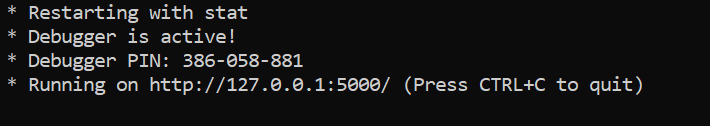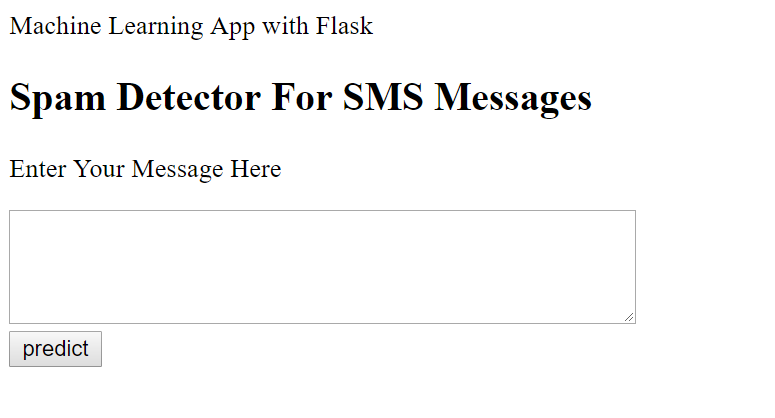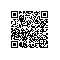使用钉钉扫一扫加入圈子
+ 订阅